Math Calculators, Lessons and Formulas

It is time to solve your math problem

mathportal.org

You included 0 formulas in your custom cheat sheet.

Cheat Sheet options

## How to generate cheat sheet ?

To create cheat sheet first you need to select formulas which you want to include in it. To select formula click at picture next to formula.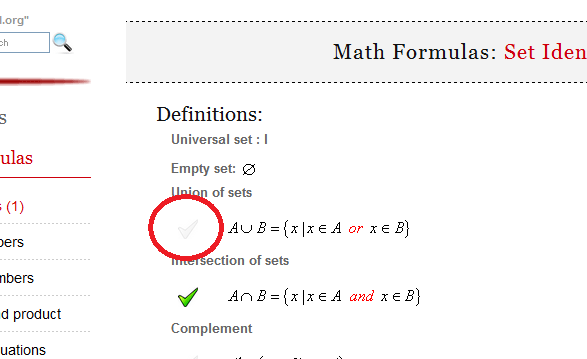You can choose formulas from different pages.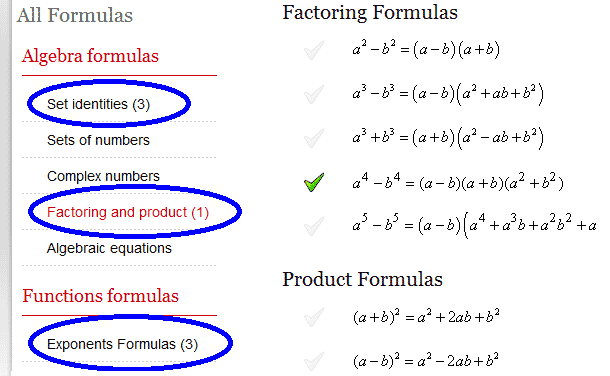After you have selected all the formulas which you would like to include in cheat sheet, click the "Generate PDF" button.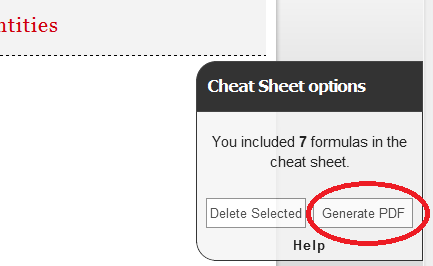# Math Formulas: Circle

### Equation of a circle

In an $x-y$ coordinate system, the circle with center $(a, b)$ and radius $r$ is the set of all points $(x, y)$ such that:

 $$(x-a)^2 + (y-b)^2 =r^2$$

Circle centered at the origin:

 $$x^2 + y^2 = r^2$$

Parametric equations

 \begin{aligned} x &= a + r\,\cos t \\ y&= b + r\,\sin t \end{aligned}

where $t$ is a parametric variable.

In polar coordinates the equation of a circle is:

 $$r^2 - 2\cdot r \cdot r_0\cdot cos(\Theta - \phi ) + r_0^2 = a^2$$

### Area of a circle

 $$A = r^2\pi$$

### Circumference of a circle

 $$C = \pi \cdot d = 2\cdot \pi \cdot r$$

### Theorems:

(Chord theorem) The chord theorem states that if two chords, $CD$ and $EF$, intersect at $G$, then:

 $$CD \cdot DG = EG \cdot FG$$

(Tangent-secant theorem) If a tangent from an external point $D$ meets the circle at $C$ and a secant from the external point $D$ meets the circle at $G$ and $E$ respectively, then

 $$DC^2 = DG \cdot DE$$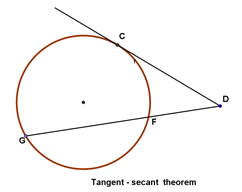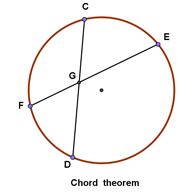(Secant - secant theorem) If two secants, $DG$ and $DE$, also cut the circle at $H$ and $F$ respectively, then:

 $$DH \cdot DG = DF \cdot DE$$

(Tangent chord property) The angle between a tangent and chord is equal to the subtended angle on the opposite side of the chord.